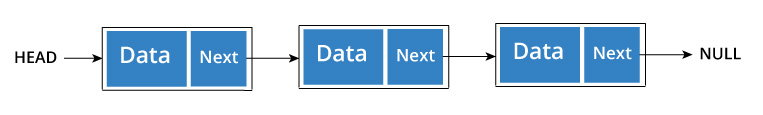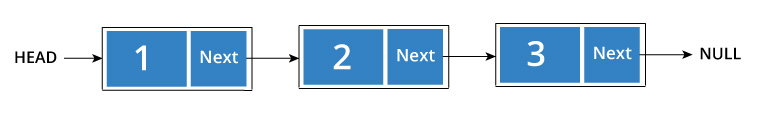#### In this tutorial, you will learn about linked list and its applications. You will also learn how to create and perform different operations on a linked list.

In a game of Treasure Hunt, you start by looking for the first clue. When you find it, instead of having the treasure, it has the location of the next clue and so on. You keep following the clues until you get to the treasure.

A linked list is similar. It is a series of connected "nodes" that contains the "address" of the next node. Each node can store a data point which may be a number, a string or any other type of data.You have to start somewhere, so we give the address of the first node a special name called HEAD.

Also, the last node in the linkedlist can be identified because its next portion points to NULL.

## How another node is referenced?

Some pointer magic is involved. Let's think about what each node contains:

• A data item
• An address of another node

We wrap both the data item and the next node reference in a struct as:

``````struct node
{
int data;
struct node *next;
};``````

Understanding the structure of a linked list node is the key to having a grasp on it.

Each struct node has a data item and a pointer to another struct node. Let us create a simple Linked List with three items to understand how this works.

``````/* Initialize nodes */
struct node *one = NULL;
struct node *two = NULL;
struct node *three = NULL;

/* Allocate memory */
one = malloc(sizeof(struct node));
two = malloc(sizeof(struct node));
three = malloc(sizeof(struct node));

/* Assign data values */
one->data = 1;
two->data = 2;
three->data=3;

/* Connect nodes */
one->next = two;
two->next = three;
three->next = NULL;

If you didn't understand any of the lines above, all you need is a refresher on pointers and structs.

In just a few steps, we have created a simple linkedlist with three nodes.The power of linkedlist comes from the ability to break the chain and rejoin it. E.g. if you wanted to put an element 4 between 1 and 2, the steps would be:

• Create a new struct node and allocate memory to it.
• Add its data value as 4
• Point its next pointer to the struct node containing 2 as data value
• Change next pointer of "1" to the node we just created.

Doing something similar in an array would have required shifting the positions of all the subsequent elements.